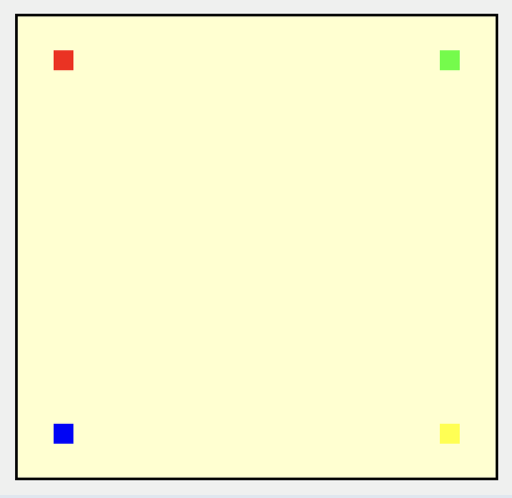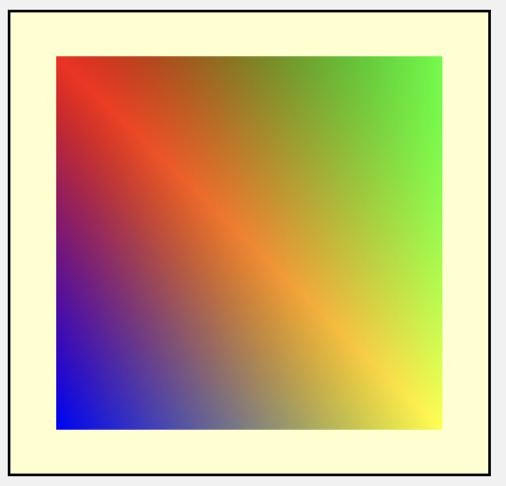# Why to pass the Camera,lighting and other info as stage_in value to Vertex function?

Hi All,

I was wondering why we need to pass the camera, transformation, world co-ordinates, tangents, bitangents, normals etc to Vertex function? The “position” attributes of-course is needed in Vertex function but other parameters are all needed only in the fragment function. In all the examples provided, I see a a generic pattern of values being passed to fragment functions via vertex function. Why cannot we pass those values directly to fragment function as a stage_in variable ? We need most of those informations in fragment functions instead of Vertex function? And do the same transformations if required in fragment function calls. Is there any reason why it has to go through vertex function?
Will there be any performance gains if we pass it directly to Fragment function instead of Vertex function?
Please let me know if there is anything which I’m not considering here.

Hi @santman and welcome to the forums! This is a great question.

Instead of looking at normals and tangents, first consider vertex color. (This may appear an odd choice because in actuality, model color is not generally rendered using vertex color, but is described by textures or material values and sent directly to the fragment function, but bear with me).

In chapter 4, “The Vertex Function”, you rendered four vertices, each with a colour attribute: `in.color`.

Points render:Two triangles render:The vertex function received in the position and the color and sent them to the rasterizer.

The rasterizer took the four vertices and converted them into two triangles, and worked out what fragments were contained inside these triangles.

The fragment function is performed for each fragment. When you pass in `in [[stage_in]]` to the fragment function, each fragment has access to the vertex attributes. But the rasterizer interpolated these attributes for each fragment, which is why you see a gradient color in the render.

If you wanted all fragments to have the same color, not a gradient, then you would pass in the color to the fragment function. This is generally how we render models. We pass the model’s material and textures directly to the fragment function.

So vertex attributes are values that should be interpolated by the rasterizer for the fragment function.

For example, you asked about normals. When normals are interpolated, just as with the color example, they will appear smooth.

This is the final render of Chapter 10, “Lighting Fundamentals”, where the sphere appears to be completely smooth:

But you can see from the wireframe, that the surface ought to be faceted with triangles.

This is the result of sending the normals through the rasterizer. You would not be able to easily calculate the value of the normal for every fragment and send it to the fragment function directly (without passing it through the rasterizer).

In the chapter 10 final sample code, you can see the effects of this interpolation if you pass the normals from the vertex function through the rasterizer with the `[[flat]]` attribute:

``````struct VertexOut {
float4 position [[position]];
float2 uv;
float3 color;
float3 worldPosition;
float3 worldNormal [[flat]];
};
``````

So in summary, every vertex attribute should go through the vertex function and be interpolated by the rasterizer and received into the fragment function with `[[stage_in]]`, and the fragment will use values interpolated between each of the three vertices that make up the triangle that covers the fragment.

Variables such as lights and camera position, with values that don’t vary with each vertex should go directly to the fragment function.

Lighting is generally done per-fragment, so that you can take advantage of the rasterizer interpolation of the vertex attributes. But it would be much quicker to do the lighting in the vertex function, as you would process it per-vertex rather than per-fragment. This is an option as described here: Per-vertex vs. per-fragment lighting but because of the artefacts, we generally do per-fragment.

I hope that helps.

2 Likes

Thanks @caroline for the great explanation. Yes this cleared my doubt. Will keep in mind the role of rasteriser in vertex functions now on1 Like